Technical Article

# Examining the Negative Resistance of a Quartz Crystal Oscillator

June 18, 2021 by Dr. Steve Arar

## This article discusses how we can examine the oscillator circuit to make sure that it has sufficient “negative resistance” or “oscillation allowance”.

In order for a crystal to start-up and sustain oscillation, the active network of the oscillator should provide a negative resistance that is large enough to compensate for the crystal losses.

### Negative Resistance Model of an Oscillator

The equivalent electrical circuit for a crystal is shown in Figure 1.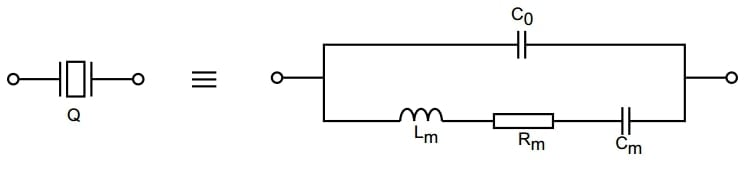##### Figure 1. Equivalent electrical circuit for a crystal. Image courtesy of STMicroelectronics.

The motional resistance (Rm) makes the circuit lossy and dissipates power. In order for the crystal to start and maintain oscillation, we need an active network to compensate for the crystal losses. The active network employs the positive feedback concept to create a negative resistance (RN) that cancels the equivalent series resistance (ESR) of the crystal at the oscillation frequency. Using the negative resistance concept, we can model the ubiquitous Pierce-Gate oscillator as shown in Figure 2.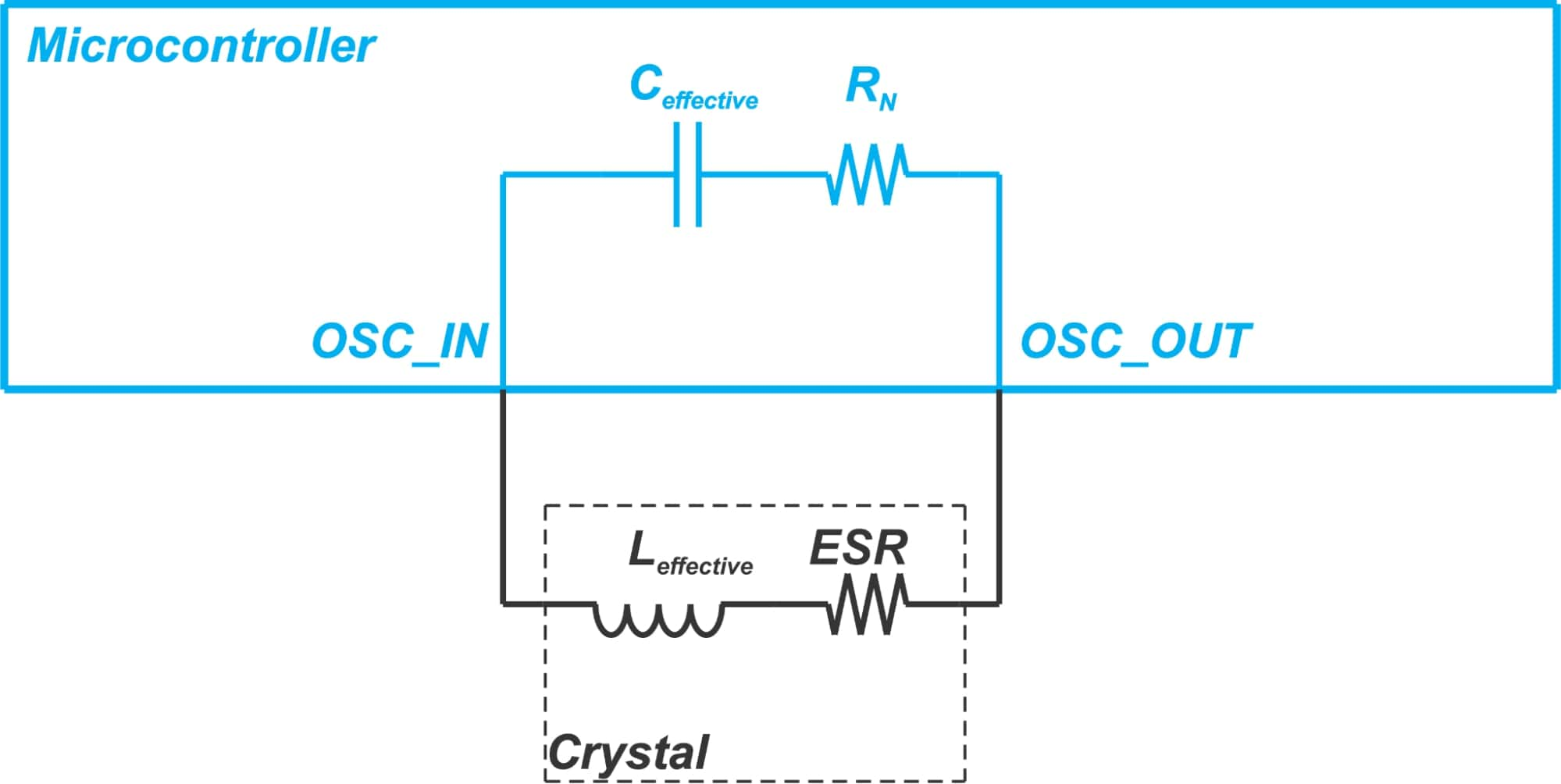##### Figure 2.

At resonance, the crystal acts as an effective inductor (Leffective) in series with an equivalent resistance (ESR). The amplifier along with its associated components can be modeled by a negative resistance (RN) in series with an effective capacitor (Ceffective). During oscillation, the negative resistance of the active network is equal to the ESR of the crystal and the reactive parts cancel each other. Hence, the oscillation criteria are:

$R_{N} = -ESR$

$X_{C effective} = -X_{L effective}$

Although |RN| is equal to ESR at a steady state, the active network should exhibit a negative resistance larger than ESR at start-up. This requirement originates from the fact that the negative resistance of the active network is a function of the oscillation amplitude. At start-up, the signal amplitude is small, and |RN| is relatively larger. As the signal amplitude increases, the negative resistance of the oscillator reduces. Finally, at a steady state, |RN| becomes equal to ESR. Hence, in order for the crystal to start oscillation, |RN| should be larger than ESR. The general rule of thumb is that |RN| should be about 5X larger than the crystal ESR:

$|R_{N}|\geq 5 \times ESR$

### Calculating the Negative Resistance of the Oscillator

We can use the simplified schematic shown in Figure 3. to model the Pierce-Gate oscillator.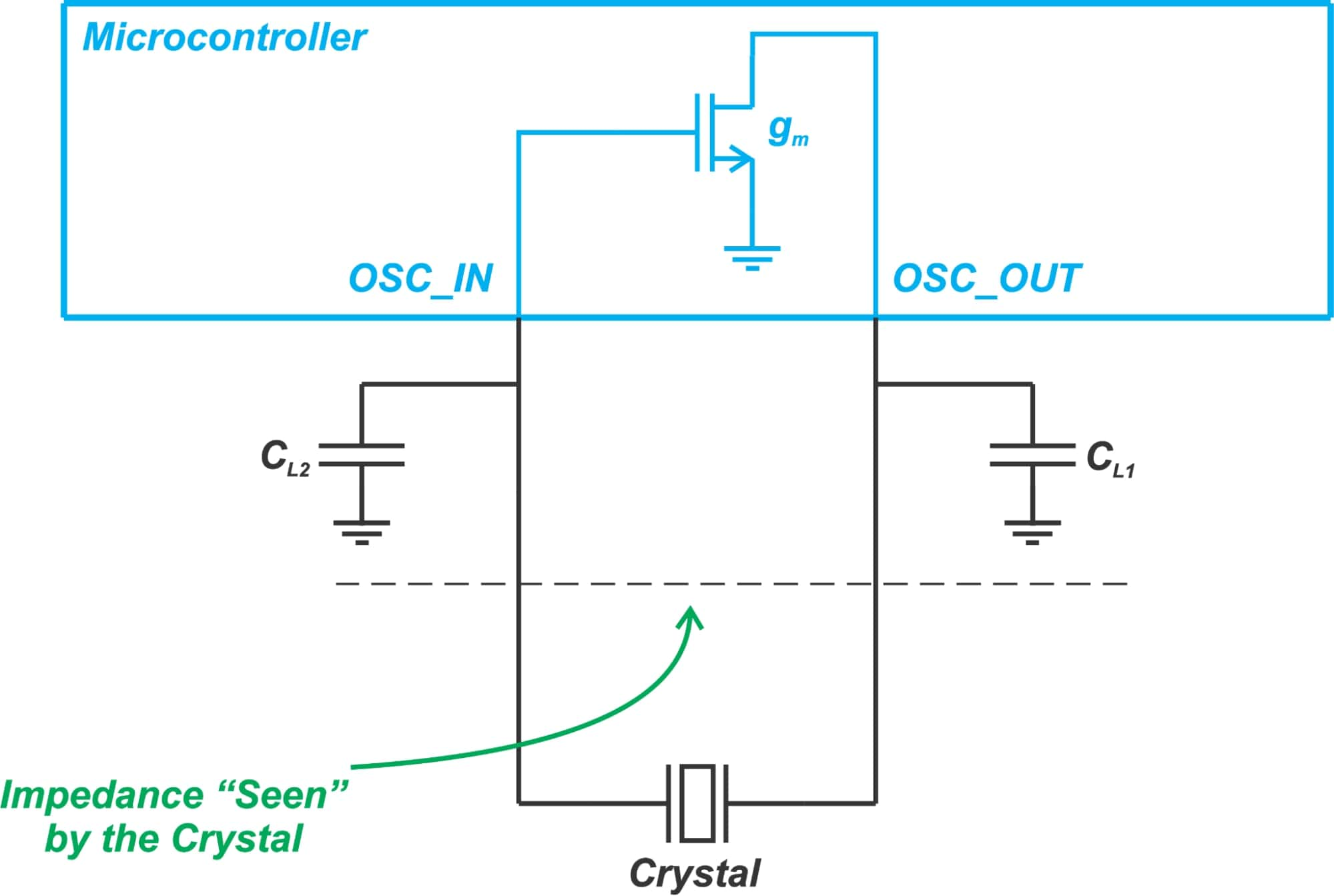##### Figure 3.

In this figure, we assume that the transistor is an ideal transconductor that models the total transconductance (gm) of the amplifier (that of both the NMOS and PMOS transistors in the actual implementation of a Pierce-Gate oscillator). To calculate the impedance “seen” by the crystal, we can replace the crystal with a test voltage source and find the ratio of the voltage across this source to its current. The impedance seen by the crystal will be:

$Z_{Effective} = \frac{1}{j2\pi f} \left(\frac{1}{C_{L1}} + \frac{1}{C_{L2}}\right) - \frac{g_m}{(2\pi f)^2C_{L1}C_{L2}}$

where f denotes the frequency of the test voltage source. The first term is the imaginary part of the impedance that should cancel the reactance of the crystal and the second term gives us the negative resistance of the active network:

$R_N = -\frac{g_m}{(2\pi f)^2C_{L1}C_{L2}}$

##### Equation 2.

As discussed above, |RN| becomes equal to the crystal ESR at a steady state. Hence, ESR determines the transconductance needed for the oscillator to start up and sustain oscillation.

Note that RN is directly proportional to the amplifier transconductance (gm) and inversely proportional to the value of the load capacitances (CL1 and CL2). Therefore, for a given RN and gm, there is an upper limit for CL1 and CL2. The lower limit of CL1 and CL2 is usually specified by the crystal manufacturer.

### Looking for a More Accurate Method

Equation 2. allows us to have a rough estimation of the required gm for a given value of negative resistance. It also gives us insight into how the negative resistance of the network changes with gm and the load capacitance. However, this equation is based on a simplified model that doesn’t take into account the circuit parasitics such as the output resistance of the amplifier, the capacitance of the MCU pins, and the stray capacitance of the PCB traces. To have a more accurate estimation of the negative resistance, some chip manufacturers give the plot of the negative resistance versus load capacitance. This method at least takes into account the non-ideal effects of the circuitry inside the chip.

Figure 4 shows the negative resistance variation for TI’s DP83xxx Ethernet PHY oscillator circuit.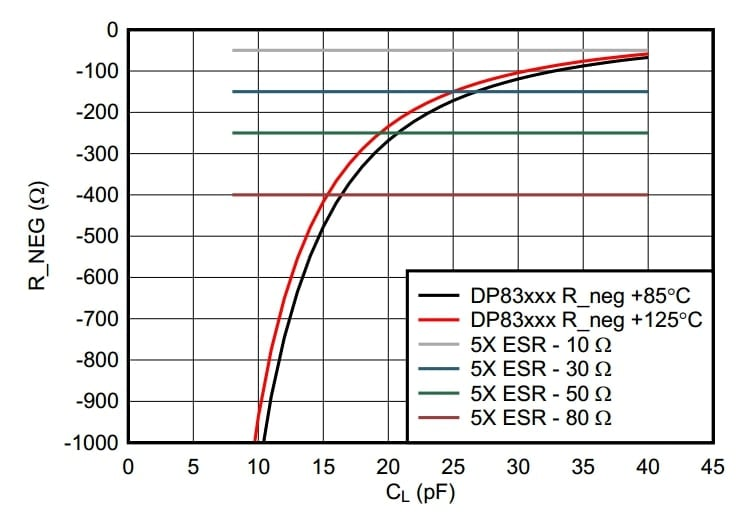##### Figure 4. Image courtesy of TI.

In this example, RN values are given for a range of load capacitance at two different temperatures (125 °C and 85 °C). The horizontal lines specify the minimum required negative resistance for some typical ESR values based on the 5X margin discussed above (Equation 1).

Although this plot can give us a more accurate estimation of the negative resistance, it cannot take all parasitics into account (e.g. the stray capacitance of the PCB traces). Besides, such plots might not be available for our intended device. A more accurate method of examining the oscillation allowance is based on measurement data from our application board that will be discussed below.

### Measuring Oscillator Negative Resistance

In order to measure the negative resistance of an oscillator, we can add a resistor in series with the crystal unit and increase the value of this resistor gradually until the oscillator is unable to start up. This is illustrated in Figure 5.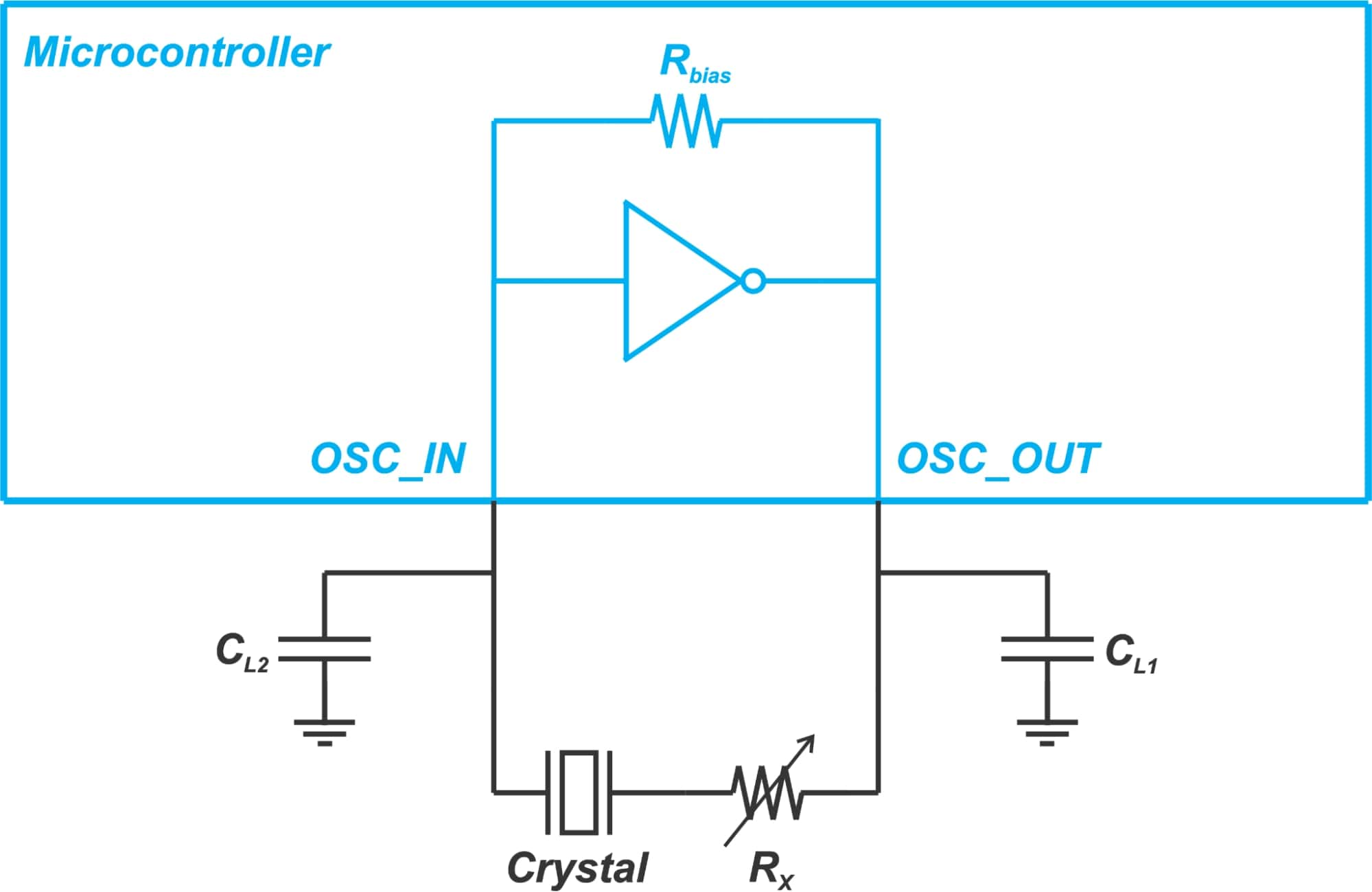##### Figure 5.

If Rx is the resistor value that prevents oscillations from occurring, the negative resistance of the oscillator is approximately equal to:

$|R_N| = ESR +R_x$

$ESR = R_m\left(1 + \frac{C_0}{C_L}\right)^2$

Figure 6 shows how this technique can be applied in practice.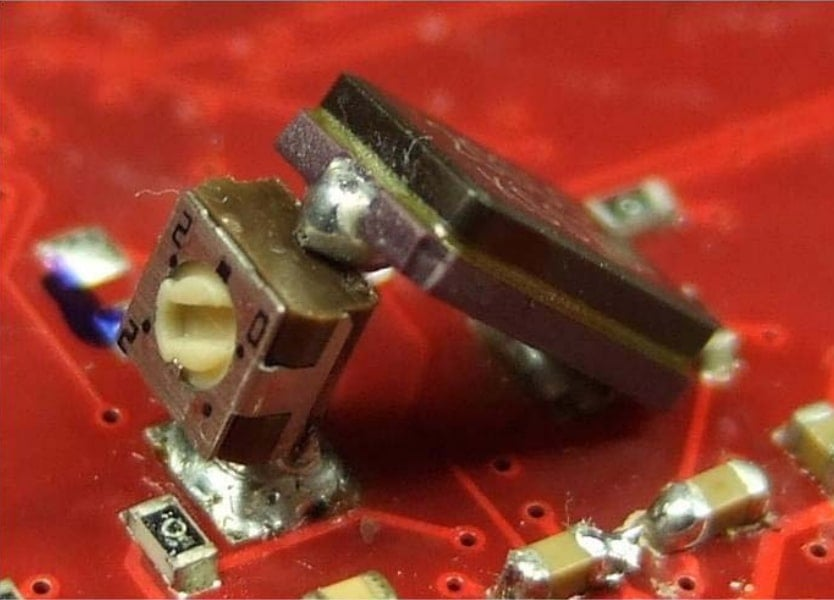##### Figure 6. Image courtesy of Infineon.

The added resistor (Rx) can be an SMD device or a potentiometer suitable for RF (Radio Frequency). In demanding applications, we might have to use a 0201 resistor to minimize the effect of parasitics on our measurements. When performing this test, it is recommended to measure the oscillation amplitude at the amplifier output (OSC_OUT in Figure 5). This node exhibits a lower impedance compared to the amplifier input (OSC_IN) and consequently, is less sensitive to the loading effect of the probe. We should also use a low capacitance probe (e.g. FET active probe) to minimize the probe loading effect.

It is worthwhile to mention that the negative resistance can decrease as we increase the temperature. That’s why we need to perform the above test both at the upper and lower limits of the operating temperature range to make sure that the circuit provides sufficient negative resistance at all temperatures. In addition to temperature, we might also need to examine the effect of supply voltage variation on oscillation reliability depending on the application requirements.

### What If You’re in the Design Stage?

The above method can be used to ensure that a given board provides a sufficient oscillation margin. However, it doesn’t give us the information we need in the design stage, e.g. the gm of the amplifier. The application note “Determining MCU Oscillator Start-up Parameters” from NXP describes how we can use the test depicted in Figure 7. to find the transconductance of the oscillator amplifier.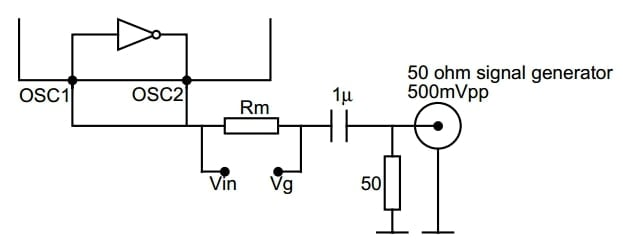##### Figure 7. Image courtesy of NXP.

The above test, along with another slightly different measurement, gives us the gm and gds parameters of the amplifier. This information can be used to ensure that our design has a sufficient oscillation margin before our prototype is available. For more information, please refer to the application note I mentioned above.